Kiekis: 0

Iš viso: 0,00

0

# Grouping of solids 1

### Grouping of solids 1

This animation demonstrates various groups of solids through examples.

Matematika

Raktiniai žodžiai

Kietųjų dalelių grupavimas, Sfera, Kūgio tipo, Cilindrinis, Piramidė, Kuboidas, Kubas, taisyklingoji prizmė, Stačiakampis, Trikampis, kietieji, rinkinys, Matematika

Susiję elementai

### Vaizdai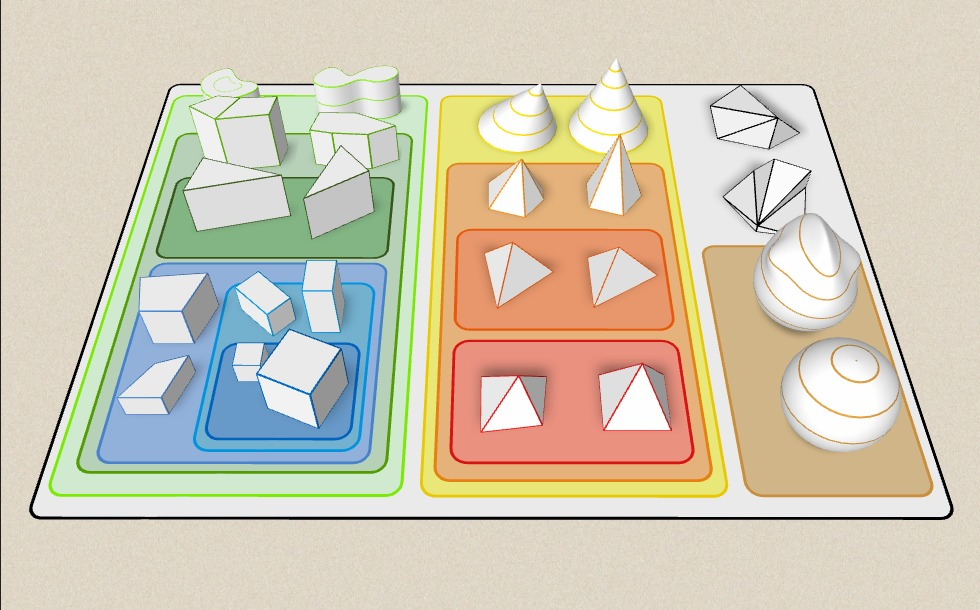### Susiję elementai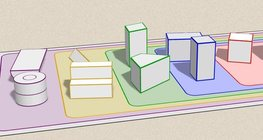#### Grouping of solids

This animation demonstrates various groups of solids through examples.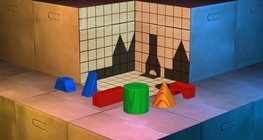This exciting and colourful game is designed to develop spatial perception. You can check your solutions using isometric views.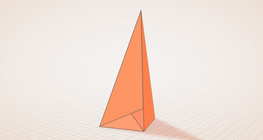#### Császár polyhedron

The Császár polyhedron is a nonconvex polyhedron with 14 triangular faces.#### Conic sections

The conic section is a plane curve that is created when a right circular cone is intersected by a plane.#### Conic solids

This animation demonstrates various types of cones and pyramids.#### Cube

This animation demonstrates the components (vertices, edges, diagonals and faces) of the cube, one of the Platonic solids.#### Cuboid

A cuboid is a polyhedron with six rectangular faces.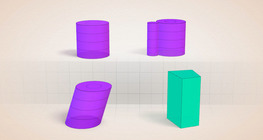#### Cylindrical solids

This animation demonstrates various types of cylindrical solids as well as their lateral surfaces.#### Euler's polyhedron formula

The theorem formulated by Leonhard Euler describes one of the basic properties of convex polyhedra.#### Grouping of solids 2

This animation demonstrates various groups of solids through examples.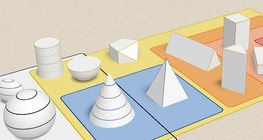#### Grouping of solids 3

This animation demonstrates various groups of solids through examples.#### Grouping of solids 4

This animation demonstrates various groups of solids through examples.#### Non-orientable surfaces

The Möbius strip and the Klein bottle are special two-dimensional surfaces with only one side.#### Optical illusion

The information gathered by the eye is misinterpreted by the brain.#### Platonic solids

This animation demonstrates the five regular three-dimensional (or Platonic) solids, the best known of which is the cube.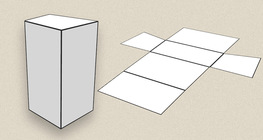#### Prisms

This animation demonstrates several types of prisms, from general to regular.#### Ratio of volumes of similar solids

This 3D scene explains the correlation between the ratio of similarity and the ratio of volume of geometric solids.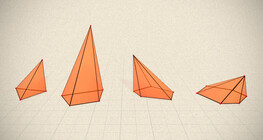#### Regular square pyramid

A regular square pyramid is a right pyramid with a square base and four triangular faces.#### Szilassi polyhedron

This special concave polyhedron was named after a Hungarian mathematician.#### Solids of revolution

Rotating a geometric shape around a line within its geometric plane as an axis results in a solid of revolution.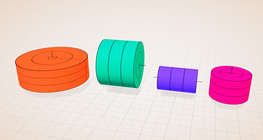#### Solids of revolution (rectangle)

Rotating a rectangle around its axes of symmetry or around its sides results in solids of revolution.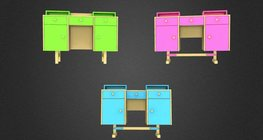#### Sophie´s desk

A game about the different views of complex objects.#### Sphere

A sphere is the set of points which are all within the same distance from a given point in space.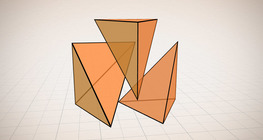#### Volume of a tetrahedron

To calculate the volume of a tetrahedron we start by calculating the volume of a prism.